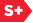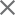### Proceedings Paper

A geometric hidden Markov tree wavelet model
Format Member Price Non-Member Price
PDF \$17.00 \$21.00

Paper Abstract

In the last few years, it has become apparent that traditional wavelet-based image processing algorithms and models have significant shortcomings in their treatment of edge contours. The standard modeling paradigm exploits the fact that wavelet coefficients representing smooth regions in images tend to have small magnitude, and that the multiscale nature of the wavelet transform implies that these small coefficients will persist across scale (the canonical example is the venerable zero-tree coder). The edge contours in the image, however, cause more and more large magnitude wavelet coefficients as we move down through scale to finer resolutions. But if the contours are smooth, they become simple as we zoom in on them, and are well approximated by straight lines at fine scales. Standard wavelet models exploit the grayscale regularity of the smooth regions of the image, but not the geometric regularity of the contours. In this paper, we build a model that accounts for this geometric regularity by capturing the dependencies between complex wavelet coefficients along a contour. The Geometric Hidden Markov Tree (GHMT) assigns each wavelet coefficient (or spatial cluster of wavelet coefficients) a hidden state corresponding to a linear approximation of the local contour structure. The shift and rotational-invariance properties of the complex wavelet transform allow the GHMT to model the behavior of each coefficient given the presence of a linear edge at a specified orientation --- the behavior of the wavelet coefficient given the state. By connecting the states together in a quadtree, the GHMT ties together wavelet coefficients along a contour, and also models how the contour itself behaves across scale. We demonstrate the effectiveness of the model by applying it to feature extraction.

Paper Details

Date Published: 13 November 2003
PDF: 7 pages
Proc. SPIE 5207, Wavelets: Applications in Signal and Image Processing X, (13 November 2003); doi: 10.1117/12.506853
Show Author Affiliations
Justin K. Romberg, Rice Univ. (United States)
Michael B. Wakin, Rice Univ. (United States)
Hyeokho Choi, Rice Univ. (United States)
Richard G. Baraniuk, Rice Univ. (United States)

Published in SPIE Proceedings Vol. 5207:
Wavelets: Applications in Signal and Image Processing X
Michael A. Unser; Akram Aldroubi; Andrew F. Laine, Editor(s)